#Function Repository Resource:

# Goldbach

Get all representations of an even integer as the sum of two primes

Contributed by: Enrique Zeleny
 ResourceFunction["Goldbach"][n] gives the even integer n represented as the sum of two primes.

## Details and Options

The Goldbach conjecture states that any even integer greater than or equal to four can be expressed as the sum of two prime numbers.
There may be many such representations.

## Examples

### Basic Examples

Some representations:

 In:=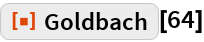Out=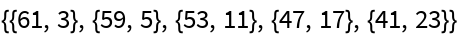In:=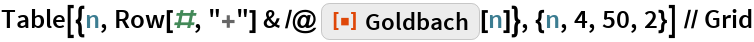Out=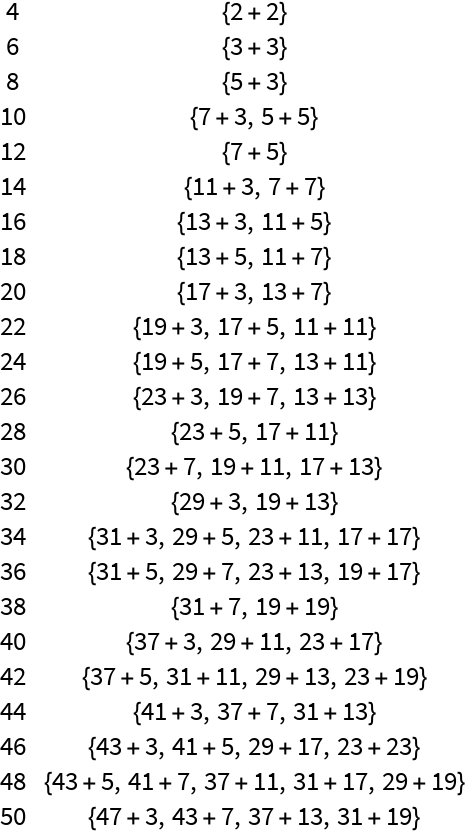The “Goldbach comet”:

 In:=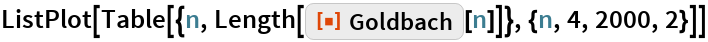Out=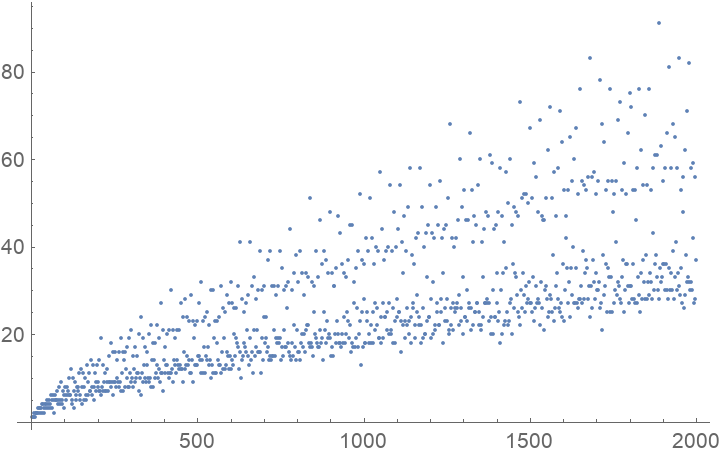The number of representations can be very large:

 In:=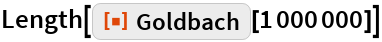Out=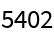Timings to compute Goldbach:

 In:=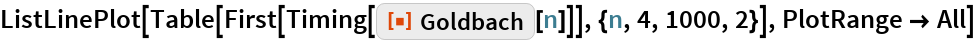Out=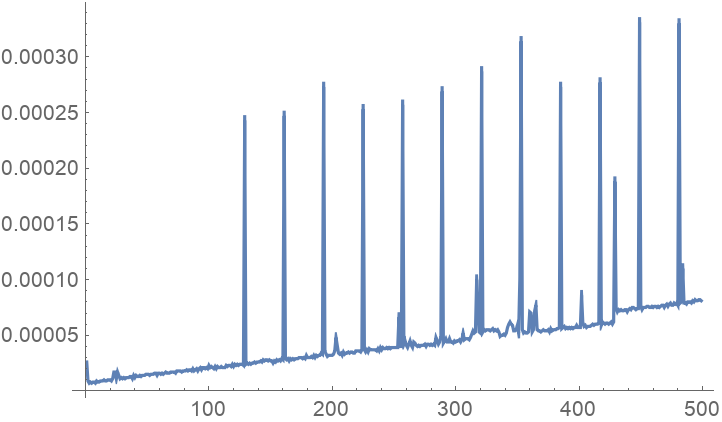## Requirements

Wolfram Language 11.3 (March 2018) or above